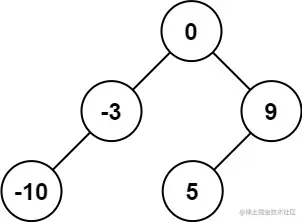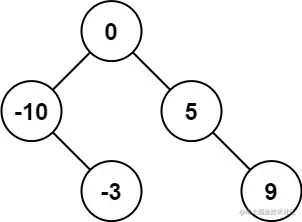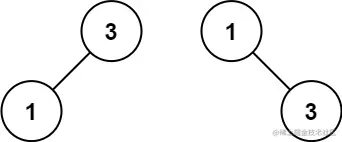# leetcode 108. Convert Sorted Array to Binary Search Tree（python）

2022-01-30 03:05:13

Little knowledge , Great challenge ！ This article is participating in “ A programmer must have a little knowledge ” Creative activities .

### describe

Given an integer array nums where the elements are sorted in ascending order, convert it to a height-balanced binary search tree.

A height-balanced binary tree is a binary tree in which the depth of the two subtrees of every node never differs by more than one.

Example 1:``````Input: nums = [-10,-3,0,5,9]
Output: [0,-3,9,-10,null,5]
Explanation: [0,-10,5,null,-3,null,9] is also accepted:
Copy code ``````Example 2:``````Input: nums = [1,3]
Output: [3,1]
Explanation: [1,3] and [3,1] are both a height-balanced BSTs.
Copy code ``````

Note:

``````1 <= nums.length <= 10^4
-10^4 <= nums[i] <= 10^4
nums is sorted in a strictly increasing order.
Copy code ``````

### analysis

According to the meaning , Is to give a list of integers nums , The elements are lists that have been sorted in ascending order . The title requires us to nums The integer in is transformed into a highly balanced binary search tree , Returns its root node .

Highly balanced binary tree is a special kind of binary tree , The depth difference between the two subtrees of each node is no more than 1.

The idea is simple , Mentioned many times before , Just use recursion to solve it directly , Just design the structure according to the meaning of the topic . Here is the sorted list nums Into a highly balanced binary tree , The key is to find the root node first , So just put nums[MID] As the root node , Then on nums[:MID] Recursion of the left subtree , Yes nums[MID+1:] Recursion of right subtree , Then we can get a highly balanced binary tree that conforms to the meaning of the question .

``````class TreeNode(object):
def __init__(self, val=0, left=None, right=None):
self.val = val
self.left = left
self.right = right
class Solution(object):
def sortedArrayToBST(self, nums):
"""
:type nums: List[int]
:rtype: TreeNode
"""
if not nums:return None
MID = len(nums)//2
root = TreeNode(nums[MID])
root.left = self.sortedArrayToBST(nums[:MID])
root.right = self.sortedArrayToBST(nums[MID+1:])
return root

Copy code ``````

### Running results

``````Runtime: 97 ms, faster than 32.83% of Python online submissions for Convert Sorted Array to Binary Search Tree.
Memory Usage: 16 MB, less than 92.78% of Python online submissions for Convert Sorted Array to Binary Search Tree.
Copy code ``````